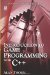# 6.9 Arithmetic Operators

## 6.9 Arithmetic Operators

Not surprisingly, the standard operators of mathematics also apply to C++ statements, namely + (addition), (subtraction), * (multiplication), and / (division). Additionally, parentheses () can be used to prioritize the evaluation of expressions over others. Consider the following.

• Statements that include the equality operator (=) are evaluated from right to left. The total value on the right-hand side is assigned to the variable on the left.

`      //answer is 0      int answer = 0;      answer = 5 + 7 + 9 - 3      //answer is now 18 `
• Variables and constants can be included in operations.

`      int number1 = 5;      int number2 = 10;      //0 is assumed if no assignment provided      int Result;      Result = number1 + number2;      //Result is 15 `
• Parentheses prioritize expressions.

`      int number1 = 5;      int number2 = 10;      //0 is assumed if no assignment provided      int Result;      Result = (number1 + number2) * 3;      //Result is 45 `Introduction to Game Programming with C++ (Wordware Game Developers Library)
ISBN: 1598220322
EAN: 2147483647
Year: 2007
Pages: 225
Authors: Alan Thorn

Similar book on Amazon

flylib.com © 2008-2017.
If you may any questions please contact us: flylib@qtcs.net